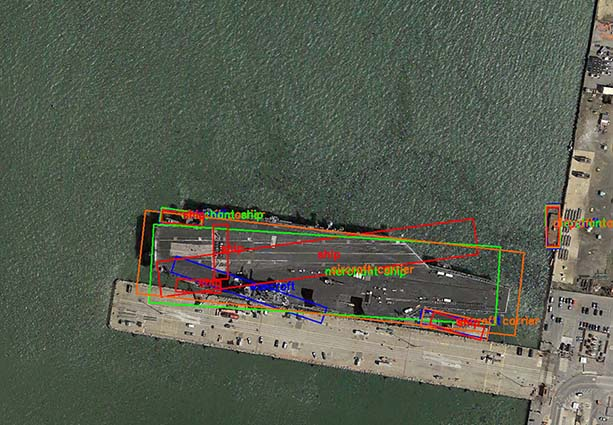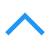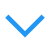# 非极大值抑制（Non-Maximum Suppression，NMS）

## NMS 在目标检测中的应用

### 人脸检测框重叠例子### 目标检测 pipline### NMS 原理(1)从最大概率矩形框F开始，分别判断A~E与F的重叠度IOU是否大于某个设定的阈值;

(2)假设B、D与F的重叠度超过阈值，那么就扔掉B、D；并标记第一个矩形框F，是我们保留下来的。

(3)从剩下的矩形框A、C、E中，选择概率最大的E，然后判断E与A、C的重叠度，重叠度大于一定的阈值，那么就扔掉；并标记E是我们保留下来的第二个矩形框。

### 代码示例

python实现的单类别nms:py_cpu_nms.py.

def py_cpu_nms(dets, thresh):
"""Pure Python NMS baseline."""
#x1、y1、x2、y2、以及score赋值
x1 = dets[:, 0]
y1 = dets[:, 1]
x2 = dets[:, 2]
y2 = dets[:, 3]
scores = dets[:, 4]

#每一个检测框的面积
areas = (x2 - x1 + 1) * (y2 - y1 + 1)
#按照score置信度降序排序
order = scores.argsort()[::-1]

keep = [] #保留的结果框集合
while order.size > 0:
i = order
keep.append(i) #保留该类剩余box中得分最高的一个
#得到相交区域,左上及右下
xx1 = np.maximum(x1[i], x1[order[1:]])
yy1 = np.maximum(y1[i], y1[order[1:]])
xx2 = np.minimum(x2[i], x2[order[1:]])
yy2 = np.minimum(y2[i], y2[order[1:]])

#计算相交的面积,不重叠时面积为0
w = np.maximum(0.0, xx2 - xx1 + 1)
h = np.maximum(0.0, yy2 - yy1 + 1)
inter = w * h
#计算IoU：重叠面积 /（面积1+面积2-重叠面积）
ovr = inter / (areas[i] + areas[order[1:]] - inter)
#保留IoU小于阈值的box
inds = np.where(ovr <= thresh)
order = order[inds + 1] #因为ovr数组的长度比order数组少一个,所以这里要将所有下标后移一位

return keep


Faster R-CNN的MATLAB实现与python版实现一致,代码在这里:nms.m.另外,nms_multiclass.m是多类别nms,加了一层for循环对每类进行nms而已.

### NMS loss### Soft-NMS

1. 线性函数

$s_i=\begin{cases} s_i, && \text{iou}(\mathcal M,b_i)<N_t \\ s_i(1-\text{iou}(\mathcal M,b_i)), && \text{iou}(\mathcal M,b_i)\ge N_t \end{cases}$

函数值不连续,在某一点的值发生跳跃.

2. 高斯函数

$s_i=s_ie^{-{\text{iou}(\mathcal M,b_i)^2}\over \sigma},\forall b_i\not \in \mathcal D$

时间复杂度同传统的greedy-NMS,为$\mathcal O(N^2)$.

ua = float((tx2 - tx1 + 1) * (ty2 - ty1 + 1) + area - iw * ih)
ov = iw * ih / ua #iou between max box and detection box

if method == 1: # linear
if ov > Nt:
weight = 1 - ov
else:
weight = 1
elif method == 2: # gaussian
weight = np.exp(-(ov * ov)/sigma)
else: # original NMS
if ov > Nt:
weight = 0
else:
weight = 1
# re-scoring 修改置信度
boxes[pos, 4] = weight*boxes[pos, 4]


Caffe C++ 版实现: makefile/frcnn

training data testing data mAP mAP@0.5 mAP@0.75 mAP@S mAP@M mAP@L Recall
Baseline D-R-FCN coco trainval coco test-dev 35.7 56.8 38.3 15.2 38.8 51.5
D-R-FCN, ResNet-v1-101, NMS coco trainval coco test-dev 37.4 59.6 40.2 17.8 40.6 51.4 48.3
D-R-FCN, ResNet-v1-101, SNMS coco trainval coco test-dev 38.4 60.1 41.6 18.5 41.6 52.5 53.8
D-R-FCN, ResNet-v1-101, MST, NMS coco trainval coco test-dev 39.8 62.4 43.3 22.6 42.3 52.2 52.9
D-R-FCN, ResNet-v1-101, MST, SNMS coco trainval coco test-dev 40.9 62.8 45.0 23.3 43.6 53.3 60.4## 其它应用

posted @ 2017-08-26 15:25  康行天下  阅读(139767)  评论(31编辑  收藏